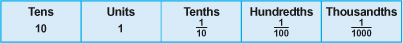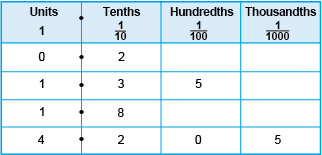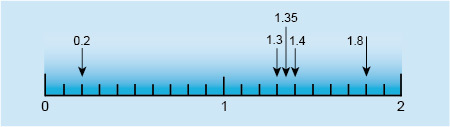Succeed with maths – Part 1

Start this free course now. Just create an account and sign in. Enrol and complete the course for a free statement of participation or digital badge if available.

Free course

# 4.1 Parts of the whole – decimal numbers

An example of a decimal number is 1.5, said as one point five. The point in the middle is called the decimal point and is used to separate the whole numbers from decimal parts of the number. The 5 in 1.5 represents the part of a whole number.

To understand how the decimal part of a number works you can start by extending the place values you have already to the right, making each one 10 times smaller. This allows you to represent numbers that are parts, or have parts of whole numbers. These are known as decimals numbers. This gives a place value table that looks like this:

Table 2 Extended place value tableThe next place value table shows a decimal point added in, showing where the whole numbers are separated from the parts of whole numbers, and some examples of decimal numbers.Figure _unit2.4.2 Figure 11 A place value table showing decimal numbers

The numbers shown in the table are:

• no units and two-tenths, written as 0.2
• one unit, three-tenths and five hundredths, written as 1.35
• one unit and eight-tenths, written as 1.8
• four units, two tenths, zero hundredths and five thousandths, written as 4.205.

As well as using a place value table you can also show decimal numbers on a number line. This may help you to visualise decimal numbers. Take a look at this number line. The intervals between the whole numbers (or units) have each been split into ten equal intervals, each one is therefore 10 times smaller than a unit, so each of these is a tenth. If each tenth had then been split into ten equal intervals, these new intervals will be hundredths, since there will be 100 of these intervals in a whole unit.Figure _unit2.4.3 Figure 12 A number line

The number line shows the following numbers expressed in decimal form:

• two-tenths, written as 0.2
• one unit and three-tenths, written as 1.3
• one unit, three-tenths and five-hundredths, written as 1.35
• one unit and four-tenths, written as 1.4
• one unit and eight-tenths, written as 1.8.

See how you get on with this next activity giving you practice at identifying the different place values.

## Activity _unit2.4.1 Activity 5 Place values

Timing: Allow approximately 10 minutes

What digit is in the hundredths position in this number?

5.603

0 is in the hundredths position.

What digit is in the thousands position?

32 657

2 is in the thousands position.

What digit is in the thousandths position?

5.8943

4 is in the thousandths position.

Now you’ve seen how you can use decimal numbers to represent numbers that include parts of a whole you’ll turn your attention to the second way that you can do this – fractions. But don’t worry you’re not going to get serious with fractions just yet – that's for Weeks 3 and 4. Here, you’re just going to think about a few everyday fractions and then see how you can relate fractions and decimal numbers to each other.